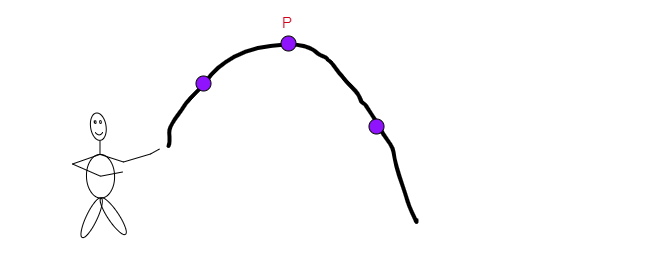(a) its acceleration
(b) its kinetic energy
(c) its potential energy
(d) its weight"
">

# A stone is thrown upwards as shown in the diagram. When it reaches P, which of the following has the greatest value for the stone?(a) its acceleration(b) its kinetic energy(c) its potential energy(d) its weight"

Here, P is the highest point of the stone. At the highest point velocity becomes zero, so the kinetic energy becomes zero and gets converted into potential energy. So, at point P its potential energy will have the greatest value.

Therefore, option (c) is corrrect.

Updated on: 10-Oct-2022

92 Views